College Physics : Snell's Law and Index of Refraction

Example Questions

Example Question #81 : College Physics

A light ray is traveling through air hits a transparent material at an angle of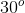from the normal. It is then refracted at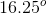. What is the speed of light in the material?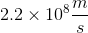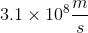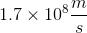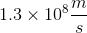Explanation:

This problem requires Snell's Law and the corresponding equation: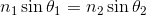We know that the index of refraction of air is: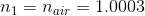We also know that: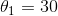and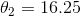Now we can plug in these values into the Snell's Law equation to find the index of refraction for the transparent material.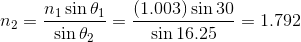Finally, we need to calculate the speed of light moving through this transparent material now that we know the index of refraction for it. To do that, we need to use this equation: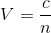Whereis the speed of light andis the index of refraction. We plug in our known values and get: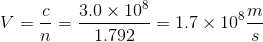All College Physics Resources